# In the Truss Shown in Figure,Compute the Forces in Each Member. - Engineering Mechanics

In the truss shown in figure,compute the forces in each member.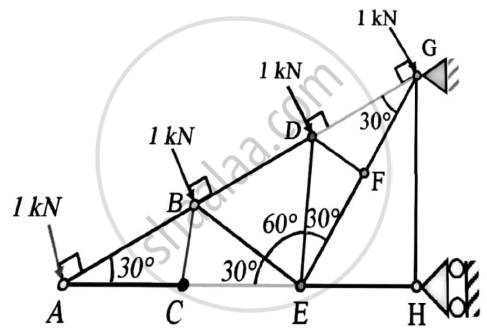#### Solution

Solution :
We can say that FD,GH and CB are zero force members in the given truss
Joint A :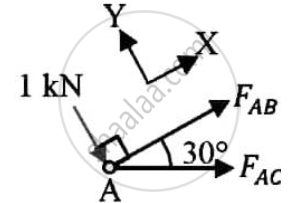Applying the conditions of equilibrium
ΣFy=0
-1 – FAC sin30 = 0
FAC = -2kN
Applying the conditions of equilibrium
ΣFx = 0
FAB + FAC cos30 = 0
FAB = 1.7321 Kn
JOINT C :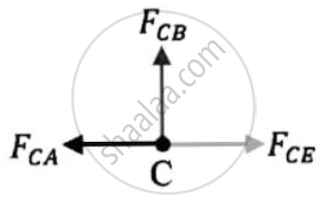Applying the conditions of equilibrium
ΣFx = 0
FCE = FCA = -2kN
JOINT B :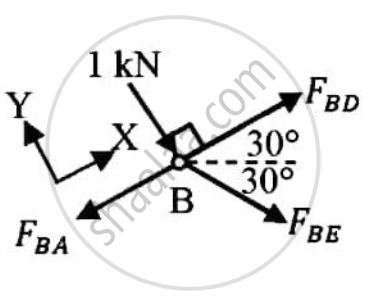Applying the conditions of equilibrium
ΣFy = 0
-1 - FBE sin60 = 0
FBE = -1.1547 kN
Applying the conditions of equilibrium
ΣFx = 0
-FBA + FBEcos60 + FBD = 0
FBD = 2.3094 kN
JOINT D :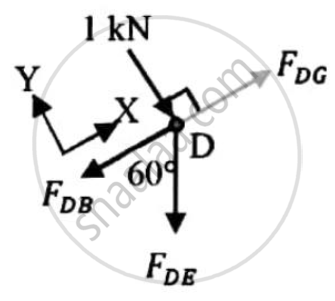Applying the conditions of equilibrium
ΣFy = 0
-1 - FDEsin60 = 0
FDE = -1.1547 kN
Applying the conditions of equilibrium
ΣFx = 0
-FDB - FDEcos60 + FDG = 0
FDG = 1.7321 kN
JOINT E :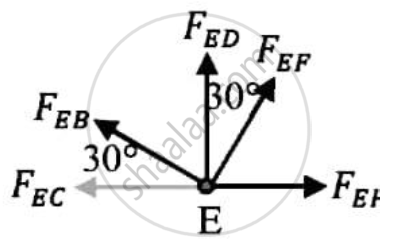Applying the conditions of equilibrium
ΣFy = 0
FED + FEFcos30 + FEBsin30 = 0
FEFcos30 = -(-1.1547)-(-1.1547) x1/2
FEF = 2kN
Joint F :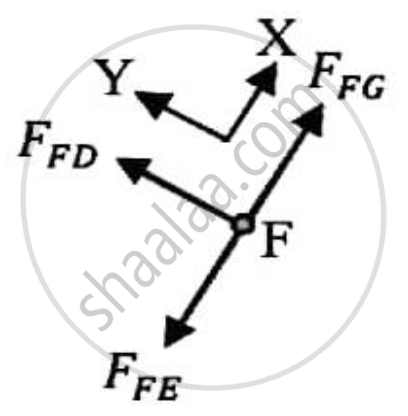Applying the conditions of equilibrium
ΣFx = 0
FFG = FFE = -2kN

 Sr.no. MEMBER MAGNITUDE OF FORCE (in kN) NATURE OF FORCE 1. AC 2 COMPRESSION 2. AB 1.7321 TENSION 3. CB 0 - 4. CE 2 COMPRESSION 5. BE 1.1547 COMPRESSION 6. BD 2.3094 TENSION 7. DE 1.1547 COMPRESSION 8.. DG 1.7321 TENSION 9. EF 2 TENSION 10. EH 4 COMPRESSION 11. FD 0 - 12. FG 2 COMPRESSION 13. GH 0 -
Concept: Analysis of Plane Trusses by Using Method of Joints
Is there an error in this question or solution?